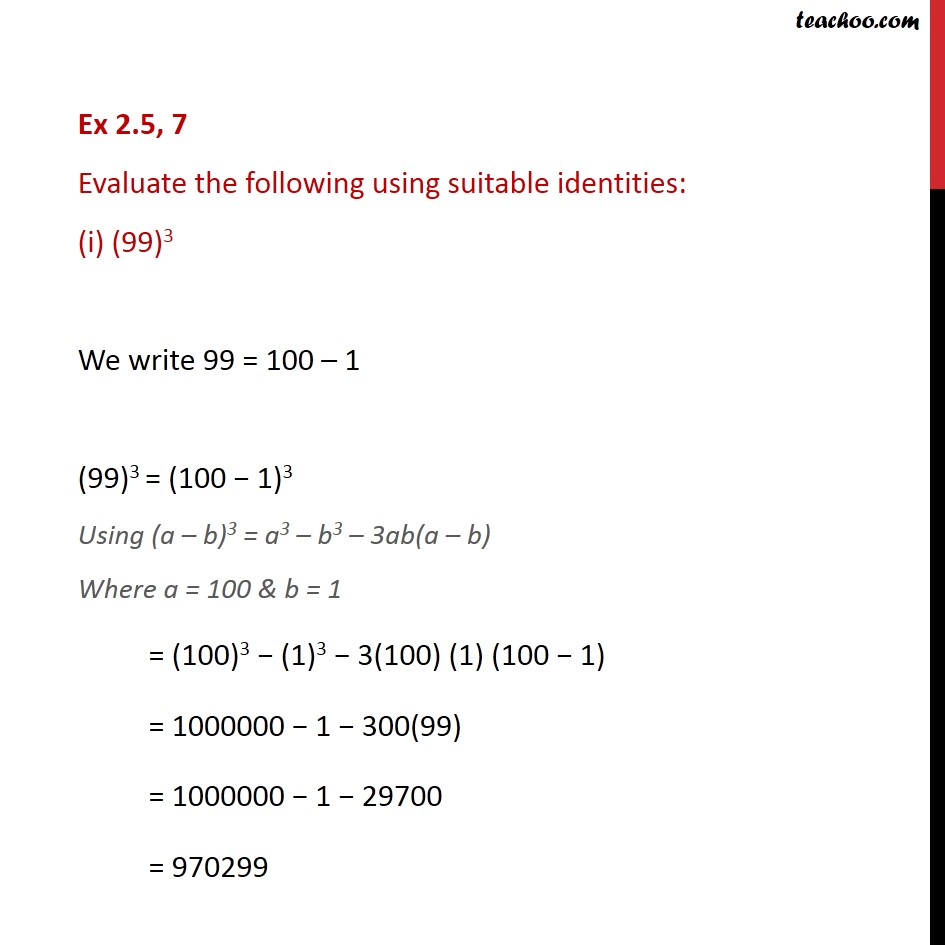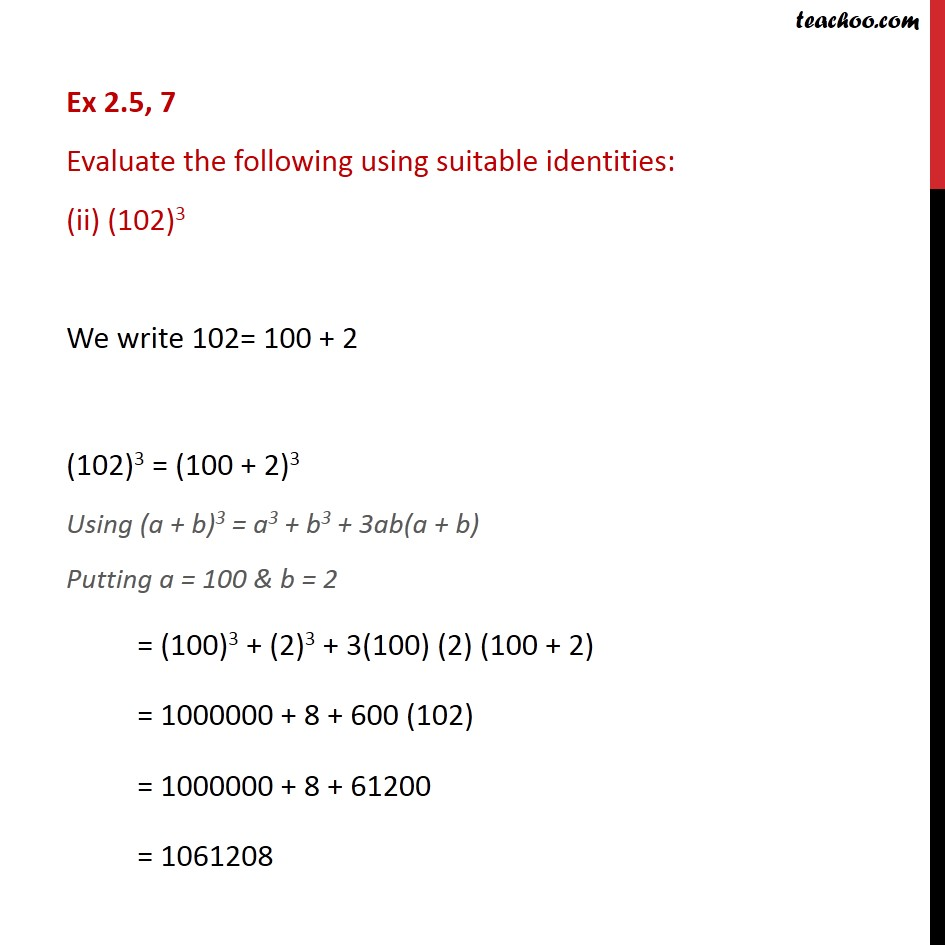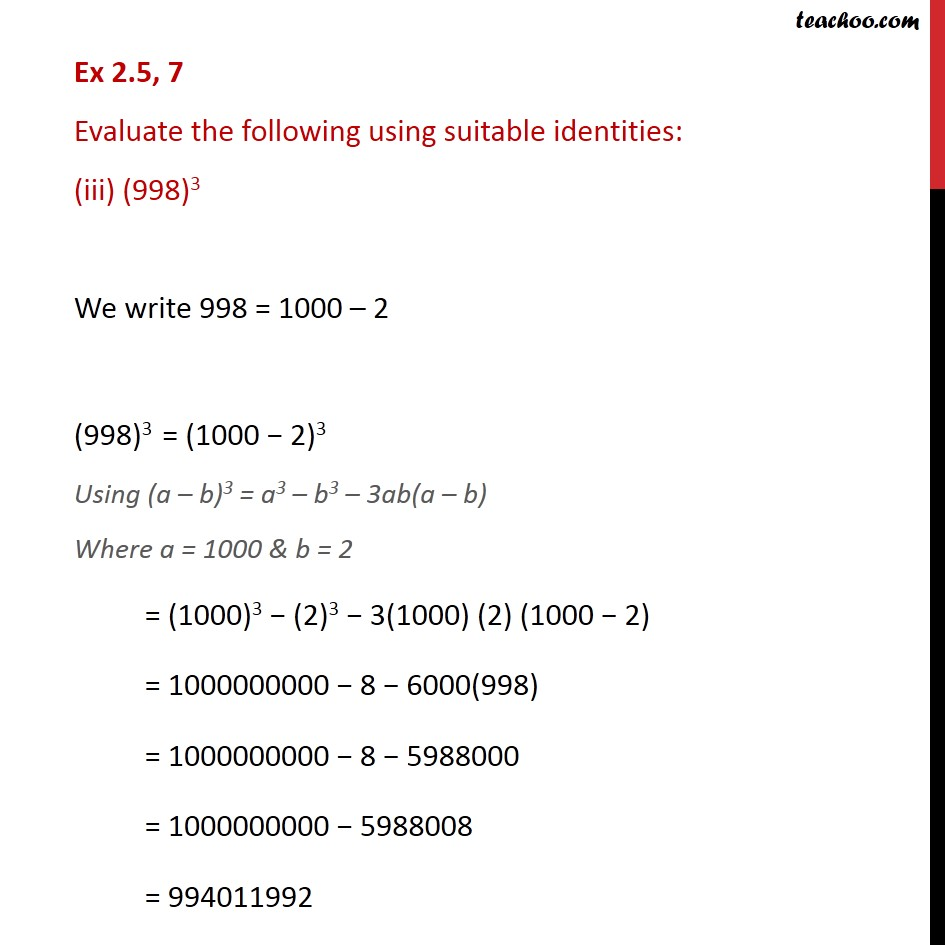1. Chapter 2 Class 9 Polynomials (Term 2)
2. Serial order wise
3. Ex 2.5

Transcript

Ex 2.5, 7 Evaluate the following using suitable identities: (i) (99)3 We write 99 = 100 – 1 (99)3 = (100 − 1)3 Using (a – b)3 = a3 – b3 – 3ab(a – b) Where a = 100 & b = 1 = (100)3 − (1)3 − 3(100) (1) (100 − 1) = 1000000 − 1 − 300(99) = 1000000 − 1 − 29700 = 970299 Ex 2.5, 7 Evaluate the following using suitable identities: (ii) (102)3 We write 102= 100 + 2 (102)3 = (100 + 2)3 Using (a + b)3 = a3 + b3 + 3ab(a + b) Putting a = 100 & b = 2 = (100)3 + (2)3 + 3(100) (2) (100 + 2) = 1000000 + 8 + 600 (102) = 1000000 + 8 + 61200 = 1061208 Ex 2.5, 7 Evaluate the following using suitable identities: (iii) (998)3 We write 998 = 1000 – 2 (998)3 = (1000 − 2)3 Using (a – b)3 = a3 – b3 – 3ab(a – b) Where a = 1000 & b = 2 = (1000)3 − (2)3 − 3(1000) (2) (1000 − 2) = 1000000000 − 8 − 6000(998) = 1000000000 − 8 − 5988000 = 1000000000 − 5988008 = 994011992

Ex 2.5